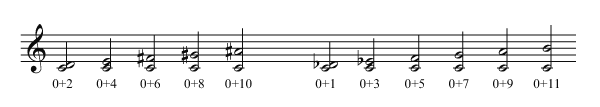Other Harmony: Sums Modulo n

Sums Modulo n

(...) Below you see at the left the intervals with an even number of semitones and at the right those with an odd number of semitones. More correctly speaking, those at the left are equivalent to 0 modulo 2, since dividing their sums (0+2, 0+4, etc.) by 2 leaves a remainder of 0, and those at the right are equivalent to 1 modulo 2, since dividing their sums (0+1, 0+3, etc.) by 2 leaves a remainder of 1. (...)